# Find a Missing Side Using the Sin Ratio

In this worksheet, students will use the Sin ratio to find the missing side of a triangle.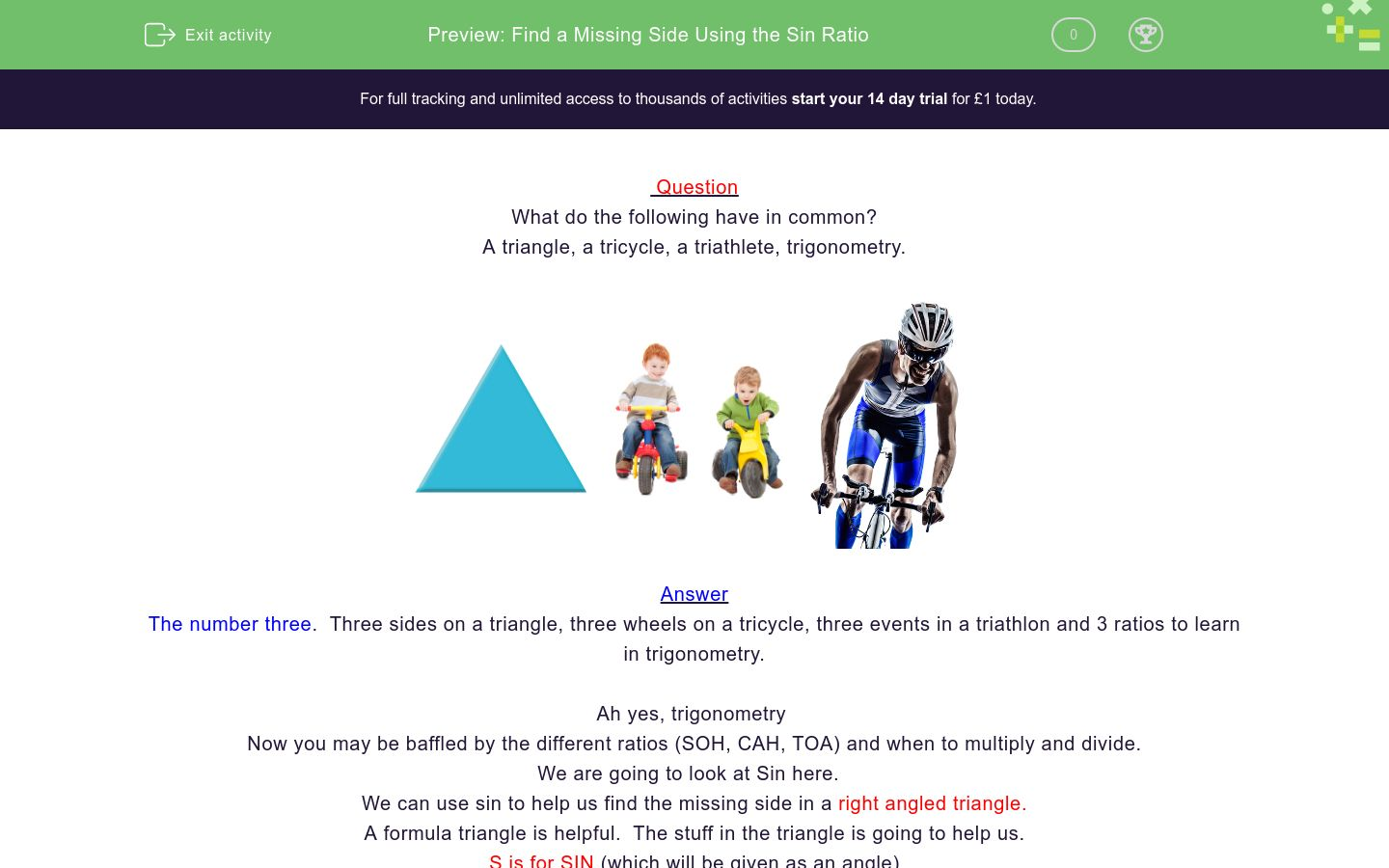Key stage:  KS 4

GCSE Subjects:   Maths

GCSE Boards:   AQA, Eduqas, OCR, Pearson Edexcel

Curriculum topic:   Geometry and Measures, Mensuration

Curriculum subtopic:   Mensuration and Calculation, Triangle Mensuration

Difficulty level:### QUESTION 1 of 10

Question

What do the following have in common?

A triangle, a tricycle, a triathlete, trigonometry.The number three.  Three sides on a triangle, three wheels on a tricycle, three events in a triathlon and 3 ratios to learn in trigonometry.

Ah yes, trigonometry

Now you may be baffled by the different ratios (SOH, CAH, TOA) and when to multiply and divide.

We are going to look at Sin here.

We can use sin to help us find the missing side in a right angled triangle.

A formula triangle is helpful.  The stuff in the triangle is going to help us.

S is for SIN (which will be given as an angle)

O is for opposite side from the angle

H is the hypotenuse

The line in the middle means divide.To use this triangle we cover up what side it is we want to find and we are left with a formula to follow.

The good old formula, where would we be without it?If we want to find the opposite side, cover up the O.  The formula we are left with is Sin(angle) x Hypotenuse.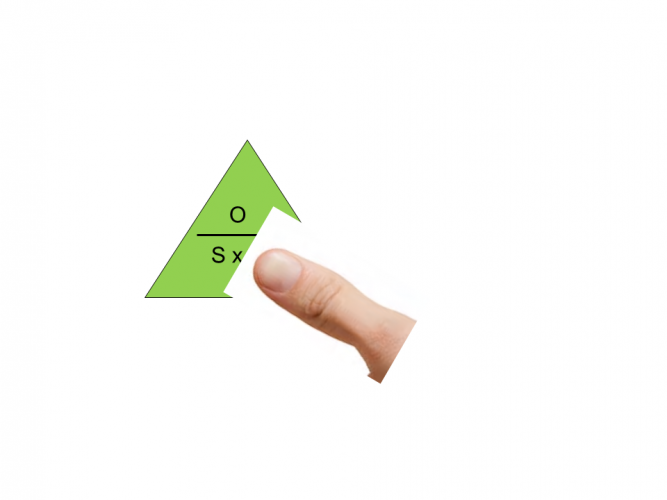If we want to find the hypotenuse, cover up the H.  The formula we are left with is opposite (O) divided by Sin(angle)Let's give it a go.

IMPORTANT NOTE: Make sure your calculator is set to degrees,  You can tell as a D should appear at the top of your calculator screen.

Example 1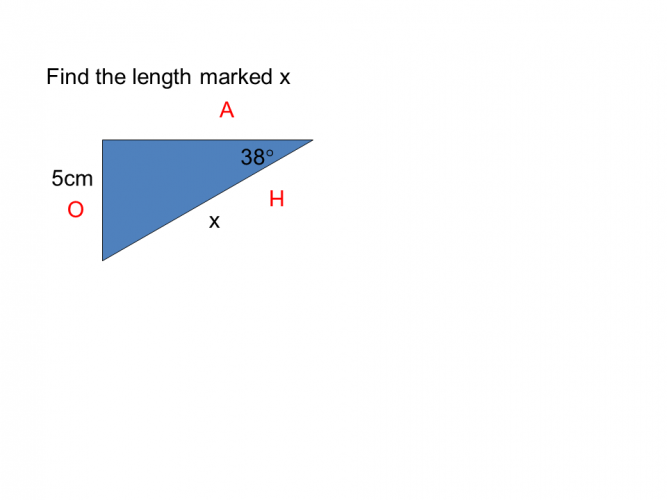1. Label the triangle

2. Find the two sides you want.  You want to find x, so we want H, and the only other side you have to help is the O.

3. Look at your formula triangle, cover up the side you want to find, which is H. (see above)

4.  We are left with O ÷ sin 38

5. In your calculator type in 5 ÷ sin 38

Example 22. Find the sides you want

3.  You should have discovered you wanted H and O

4. Use your formula triangle and cover up O the side you want to find

5. The formula you are left with is Sin 27 x 10 = 4.54 to 2 decimal places

Don't you think it quite fitting that a trigonometry question has a formula triangle to help us?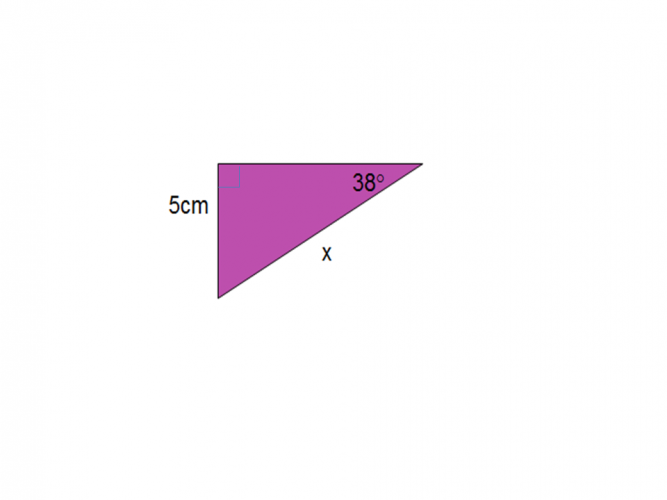Find the length marked x.

Answer has been rounded to 2 decimal places

8.45 cm

8.38 cm

8.12 cm

8.65 cm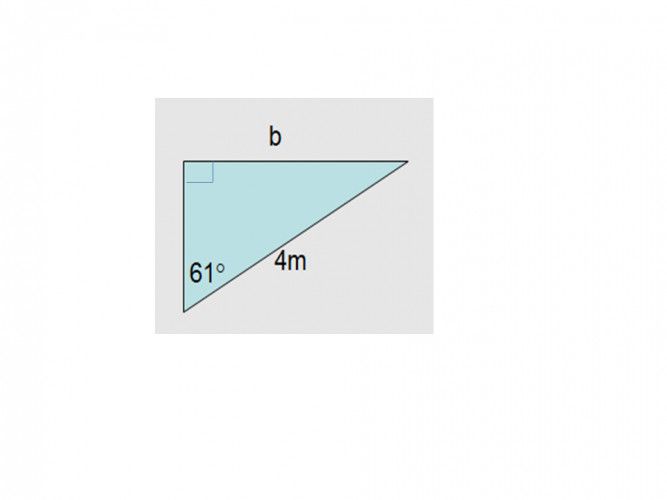Find the value of bsin x hypotenuse opposite divided by sin 4.12 cm 5.12 cm 6.12 cm The formula I need is The value of x issin x hypotenuse opposite divided by sin 4.12 cm 5.12 cm 6.12 cm The formula I need is The value of x isFind the length of x

8.25 cm

6.75 cm

7.95 cm

9.55 cmSin x hypotenuse opposite divided by sin 8.48 cm 16.97 cm 17.83 cm The formula I need is the value of x isSin x hypotenuse opposite divided by sin 8.48 cm 16.97 cm 17.83 cm The formula I need is the value of x isFind the length of x.Find the value of x.

Answer has been rounded to 2 decimal places (2 d.p)

13.47 cm

12.82 cm

14.32 cm

15.64 cm

• Question 1Find the length marked x.

Answer has been rounded to 2 decimal places

8.12 cm
EDDIE SAYS
The tricky part of the question is labelling your triangle correctly. Think of the labelling as a sin post. You should have discovered that you needed the opposite divided by Sin 38. 5 ÷ sin 38 = 8.12 cm (to 2 d.p)
• Question 2Find the value of b

3.5
EDDIE SAYS
How are you going with the labelling of the triangle? It can get tricky I know. You should have discovered that you needed the Sin x the hypotenuse. Sin 61 x 4 = 3.49 (3.5 to 1 d.p) sin sational!!
• Question 3sin x hypotenuse opposite divided by sin 4.12 cm 5.12 cm 6.12 cm The formula I need is The value of x is
EDDIE SAYS
Labelling the triangle correctly is key to unlocking he correct formula. You should have discovered you needed sin and the hypotenuse. sin 47 x 7 = 5.12 cm to 2 decimal places
• Question 4EDDIE SAYS
How are you getting on using the formula triangle? For this one you needed to find the hypotenuse so the formula was 5 ÷ sin 38 = 8.12 cm sin tillating stuff huh?
• Question 5Find the length of x

7.95 cm
EDDIE SAYS
Are you getting used to this now? This was a multiplication as we wanted to find the opposite. Sin 32 x 15 = 7.95 cm to (2 d.p). When finding a side we always need the angle, it is just a case of deciding if we multiply or divide with it.
• Question 64.05
EDDIE SAYS
Sometimes we are given the information in written format such as this. Find side ED. You can see that it is opposite therefore sin 35.3 x 7 = 4.05 cm (to 2 d.p)
• Question 7Sin x hypotenuse opposite divided by sin 8.48 cm 16.97 cm 17.83 cm The formula I need is the value of x is
EDDIE SAYS
To be honest once you can label your triangle correctly you can leave the rest to your scientific calculator. What a great friend it is at a time like this. 12 ÷ sin 45 = 16.97 cm
• Question 8EDDIE SAYS
Calculators rock. The only thing you have to be careful of, is rounding to the correct number of decimal places. sin33 x 21 = 11.44 cm (to 2 d.p)
• Question 9Find the length of x.

77.12
EDDIE SAYS
Nearly there. I hope you haven't found this to tri-ing!! opposite ÷ sin 33 42 ÷ sin 33 = 77.12 (to 2 d.p)
• Question 10Find the value of x.

Answer has been rounded to 2 decimal places (2 d.p)

13.47 cm
EDDIE SAYS
Are you ready to sin out now? One final check though 12 ÷ sin 63 = 13.47 cm (to 2 d.p)
---- OR ----

Sign up for a £1 trial so you can track and measure your child's progress on this activity.

### What is EdPlace?

We're your National Curriculum aligned online education content provider helping each child succeed in English, maths and science from year 1 to GCSE. With an EdPlace account you’ll be able to track and measure progress, helping each child achieve their best. We build confidence and attainment by personalising each child’s learning at a level that suits them.

Get started